Courses

# Previous Year Questions (2014-19) - The p-Block Elements (Group 15, 16, 17 and 18) Notes | EduRev

## NEET : Previous Year Questions (2014-19) - The p-Block Elements (Group 15, 16, 17 and 18) Notes | EduRev

The document Previous Year Questions (2014-19) - The p-Block Elements (Group 15, 16, 17 and 18) Notes | EduRev is a part of the NEET Course Chemistry 28 Years Past year papers for NEET/AIPMT Class 12.
All you need of NEET at this link: NEET

Q.1. Match the Xenon compounds in Column-I with its structure in Column-II and assign the correct code:    (2019)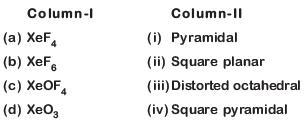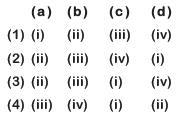A: (1)
B: (2)
C: (3)
D: (4)
Ans:
B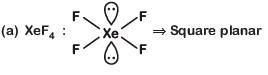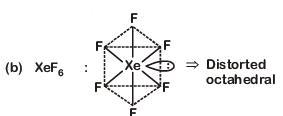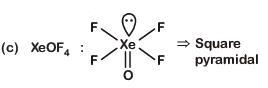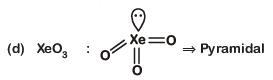Q.2. Which is the correct thermal stability order for H2E (E = O, S, Se, Te and Po)?    (2019)
A: H2S < H2O < H2Se < H2Te < H2Po
B: H2O < H2S < H2Se < H2Te < H2Po
C: H2Po < H2Te < H2Se < H2S < H2O
D: H2Se < H2Te < H2Po < H2O < H2S
Ans:
C
On going down the group thermal stability order for H2E decreases because H–E bond energy decreases
∴ Order of stability would be:-
H2Po < H2Te < H2Se < H2S < H2O

Q.3. Which of the following statements is not true for halogens ?    (2018)
A: All form monobasic oxyacids.
B: All are oxidizing agents.

C: All but fluorine show positive oxidation states.
D: Chlorine has the highest electron-gain enthalpy.
Ans:
C
Due to high electronegativity and small size, F forms only one oxoacid, HOF known as Fluoric (I) acid. Oxidation number of F is +1 in HOF.

Q.4. In the structure of ClF3, the number of lone pairs of electrons on central atom 'Cl' is    (2018)
A: one
B: two
C: three
D: four

Ans: B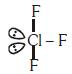2 lone pairs at equitorial position.

Q.5. Match the interhalogen compounds of column-I with the geometry in column II and assign the correct code.    (2017)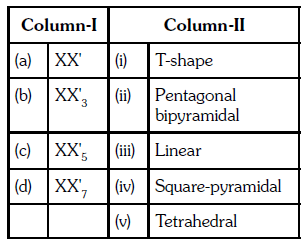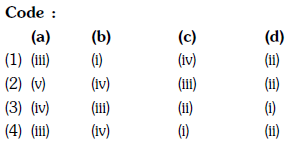A: (1)
B: (2)
C: (3)
D: (4
Ans:
A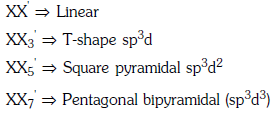Q.6. In which pair of ions both the species contain S–S bond?    (2017)
A: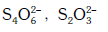B: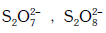C: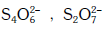D: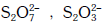Ans:
A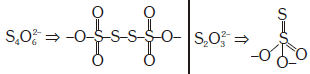Q.7. When copper is heated with conc. HNO3 it produces :    (2016)
A: Cu(NO3)2 and N2O
B: Cu(NO3)2 and NO2
C: Cu(NO3)2 and NO
D: Cu(NO3)2 , NO and NO2
Ans:
B
Nitric acid acts as an oxidising agent while reacting with copper.
i) when copper reacts with dilute nitric acid it forms,
3Cu + 4HNO3(dilute) → 3Cu(NO3)2 +2NO +4H2O
ii) When copper reacts with concentrated nitric acid it forms,
Cu +4HNO3(conc.) → Cu(NO3)2 +NO2 +2H2O

Q.8. Which is the correct statement for the given acids?    (2016)
A: Phosphinic acid is a diprotic acid while phosphonic acid is a monoprotic acid.
B: Phosphinic acid is a monoprotic acid while phosphonic acid is a diprotic acid.
C: Both are diprotic acids.
D: Both are triprotic acids.
Ans:
B
Phosphinic acid is a monoprotic acid while phosphonic acid is a diprotic acid.
Phosphinic acid-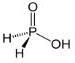Phosphonic acid-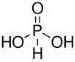Due to the presence of one replaceable proton in phosphinic acid, it is monoprotic acid and due to the presence of two replaceable proton in phosphinic acid, it is diprotic acid.

Q.9. Among the following, the correct order of acidity is :    (2016)
A: HCIO4 < HCIO2 < HCIO < HCIO3
B: HCIO3 < HCIO4 < HCIO2 < HCIO
C: HCIO < HCIO2 < HCIO3 < HCIO4
D: HCIO2 < HCIO < HCIO3 < HCIO4
Ans:
C
As oxidation number of central atom increases, acidic nature increases.
HClO < HClO2 < HClO3 < HClO4

Q.10. The product obtained as a result of a reaction of nitrogen with CaCis :    (2016)
A: Ca2CN
B: Ca(CN)2
C: CaCN
D: CaCN3
Ans:
B
When calcium carbide reacts with nitrogen under high temperature, it forms calcium cyanamide which is also called nitrolim.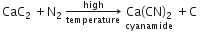Q.11. Which one of the following orders is correct for the bond dissociation enthalpy of halogen molecules?    (2016)
A: F2 > Cl2 > Br2 > I2
B: I2 > Br2 > CI2 > F2
C: CI2 > Br2 > F2 > I2
D: Br2 > I2 > F2 > CI2
Ans:
C
Bond dissociation energies of halogen family decrease down the group as the size of the atom increases. The bond dissociation energy of fluorine, is, however, lower than those of chlorine and bromine because of interelectronic repulsions present in the small atom of fluorine.
Hence bond energy decreases in the order Cl2 > Br2 > F2 > I2

Q.12. Match the compound given in column I with the hybridization and shape given in column II and mark the correct option.    (2016)
Column-I                Column-II
(a) XeF6            (i) distorted octahedral
(b) XeO3           (ii) square planar
(c) XeOF4         (iii) pyramidal
(d) XeF4            (iv) square pyramidal
Code :
(a) (b) (c) (d)
A: (iv) (i) (ii) (iii)
B: (i) (iii) (iv) (ii)
C: (i) (ii) (iv) (iii)
D: (iv) (iii) (i) (ii)
Ans:
B
(i) (iii) (iv) (ii)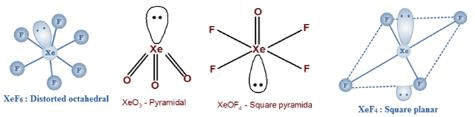Q.13. Which of the following species contain equal number of σ - and π - bonds ?    (2015)
A: CH2(CN)2
B: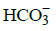C: XeO4
D:(CN)2
Ans:
C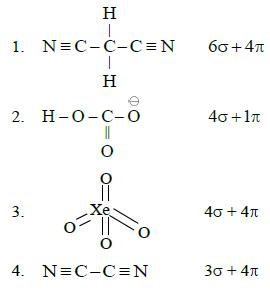Q.14. Nitrogen dioxide and sulphur dioxide have some properties in common. Which property is shown by one of these compounds, but not by the other ?    (2015)
A: is used as a food-preservative
B: forms 'acid-rain'
C: is a reducing agent
D: is soluble in water
Ans:
A
SO2 is used in the manufacture of sodium bisulphate (NaHSO3) which is used as preservatives for jams, jellies and squashes.

Q.15. Which of the following pairs of ions are isoelectronic and isostructural ?    (2015)
A: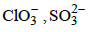B: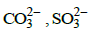C: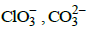D: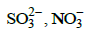Ans:
A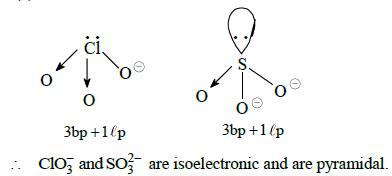Q.16. Maximum bond angle at nitrogen is present in which of the following ?    (2015)
A: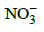B: NO2
C: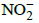D: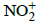Ans: D
In all of the four molecules NO2- and NO2 have one lone pair thus bond angle is less than 1200 in the case of NO2- and more than 120in the case of NO2 but in the NO3- there is no lone pair hence all are bonding pairs leading to an ideal bond angle of 1200.
In NO2+, the is no lone pair only bond pair exist thus leading a bond angle is 1800.

Q.17. Acidity of diprotic acids in aqueous solutions increases in the order :    (2014)
A: H2Te < H2S < H2Se
B: H2Se < H2Te < H2S
C: H2S < H2Se < H2Te
D: H2Se < H2S < H2Te
Ans:
C
Acidic strength of hydrides increases as the size
of central atom increases which weakens the
M- H bond. Since, the size increases from S to Te
thus acidic strength follows the order.
H2S<H2Se<H2Te
Acidic nature ∝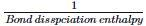S to Te size increases, bond dissociation enthalpy
decreases and acidic nature increases.

Offer running on EduRev: Apply code STAYHOME200 to get INR 200 off on our premium plan EduRev Infinity!

,

,

,

,

,

,

,

,

,

,

,

,

,

,

,

,

,

,

,

,

,

,

,

,

,

,

,

;